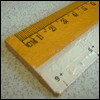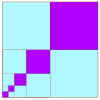# Resources tagged with: Limits of Sequences

Filter by: Content type:
Age range:
Challenge level:

### There are 14 results

Broad Topics > Patterns, Sequences and Structure > Limits of Sequences### Slide

##### Age 16 to 18 Challenge Level:

This function involves absolute values. To find the slope on the slide use different equations to define the function in different parts of its domain.### Squareness

##### Age 16 to 18 Challenge Level:

The family of graphs of x^n + y^n =1 (for even n) includes the circle. Why do the graphs look more and more square as n increases?### Small Steps

##### Age 16 to 18 Challenge Level:

Two problems about infinite processes where smaller and smaller steps are taken and you have to discover what happens in the limit.### How Does Your Function Grow?

##### Age 16 to 18 Challenge Level:

Compares the size of functions f(n) for large values of n.### Ruler

##### Age 16 to 18 Challenge Level:

The interval 0 - 1 is marked into halves, quarters, eighths ... etc. Vertical lines are drawn at these points, heights depending on positions. What happens as this process goes on indefinitely?### Climbing Powers

##### Age 16 to 18 Challenge Level:

$2\wedge 3\wedge 4$ could be $(2^3)^4$ or $2^{(3^4)}$. Does it make any difference? For both definitions, which is bigger: $r\wedge r\wedge r\wedge r\dots$ where the powers of $r$ go on for ever, or. . . .### Little and Large

##### Age 16 to 18 Challenge Level:

A point moves around inside a rectangle. What are the least and the greatest values of the sum of the squares of the distances from the vertices?### Continued Fractions I

##### Age 14 to 18

An article introducing continued fractions with some simple puzzles for the reader.### Continued Fractions II

##### Age 16 to 18

In this article we show that every whole number can be written as a continued fraction of the form k/(1+k/(1+k/...)).### Approximating Pi

##### Age 14 to 18 Challenge Level:

By inscribing a circle in a square and then a square in a circle find an approximation to pi. By using a hexagon, can you improve on the approximation?### A Swiss Sum

##### Age 16 to 18 Challenge Level:

Can you use the given image to say something about the sum of an infinite series?### Archimedes and Numerical Roots

##### Age 14 to 16 Challenge Level:

The problem is how did Archimedes calculate the lengths of the sides of the polygons which needed him to be able to calculate square roots?### Infinite Continued Fractions

##### Age 16 to 18

In this article we are going to look at infinite continued fractions - continued fractions that do not terminate.### Summing Geometric Progressions

##### Age 14 to 18 Challenge Level:

Watch the video to see how to sum the sequence. Can you adapt the method to sum other sequences?